# reflector

Create reflector-backed antenna

## Description

The `reflector` object is a reflector-backed antenna on the X-Y-Z plane. The default reflector antenna uses a dipole as an exciter. The feed point is on the exciter.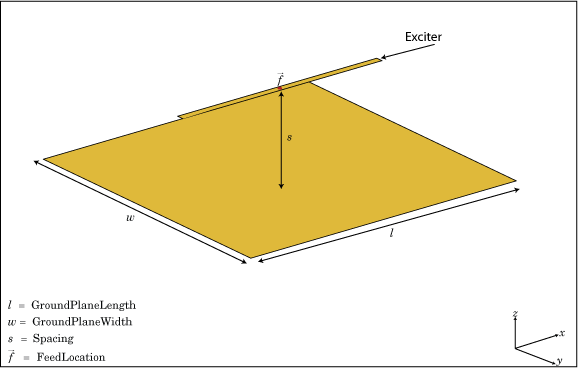## Creation

### Syntax

``rf = reflector``
``rf = reflector(Name,Value)``

### Description

``` `rf = reflector` creates a reflector backed antenna located in the X-Y-Z plane. By default, dimensions are chosen for an operating frequency of 1 GHz.```

example

``` `rf = reflector(Name,Value)` creates a reflector backed antenna, with additional properties specified by one or more name-value pair arguments. `Name` is the property name and `Value` is the corresponding value. You can specify several name-value pair arguments in any order as `Name1`, `Value1`, `...`, `NameN`, `ValueN`. Properties not specified retain their default values.```

## Properties

expand all

Antenna type used as an exciter, specified as any single-element antenna object. Except reflector and cavity antenna elements, you can use any of the antenna elements or array elements in the Antenna Toolbox™ as an exciter.

Example: `'Exciter',horn`

Example: `ant.Exciter = horn`

Example: ```ant.Exciter = linearArray('patchMicrostrip')```

Type of dielectric material used as a substrate, specified as an object. For more information see, `dielectric`. For more information on dielectric substrate meshing, see Meshing.

Note

The substrate dimensions must be equal to the groundplane dimensions.

Example: ```d = dielectric('FR4'); 'Substrate',d```

Example: ```d = dielectric('FR4'); rf.Substrate = d```

Reflector length along the x-axis, specified a scalar in meters. By default, ground plane length is measured along the x-axis. Setting `'GroundPlaneLength'` to`Inf`, uses the infinite ground plane technique for antenna analysis. You can also set the `'GroundPlaneLength'` to zero.

Example: `'GroundPlaneLength',3`

Data Types: `double`

Reflector width along the y-axis, specified as a scalar in meters. By default, ground plane width is measured along the y-axis. Setting `'GroundPlaneWidth'` to`Inf`, uses the infinite ground plane technique for antenna analysis. You can also set the `'GroundPlaneWidth'` to zero.

Example: `'GroundPlaneWidth',2.5`

Data Types: `double`

Distance between the reflector and the exciter, specified as a scalar in meters. By default, the exciter is placed along the x-axis.

Example: `'Spacing',7.5e-2`

Data Types: `double`

Type of the metal used as a conductor, specified as a metal material object. You can choose any metal from the `MetalCatalog` or specify a metal of your choice. For more information, see `metal`. For more information on metal conductor meshing, see Meshing.

Example: ```m = metal('Copper'); 'Conductor',m```

Example: ```m = metal('Copper'); ant.Conductor = m```

Lumped elements added to the antenna feed, specified as a lumped element object handle. For more information, see `lumpedElement`.

Example: `'Load',lumpedelement`. `lumpedelement` is the object handle for the load created using `lumpedElement`.

Example: ```rf.Load = lumpedElement('Impedance',75)```

Create probe feed from backing structure to exciter, specified as `0` or `1`. By default, probe feed is not enabled.

Example: `'EnableProbeFeed',1`

Data Types: `double`

Tilt angle of the antenna, specified as a scalar or vector with each element unit in degrees. For more information, see Rotate Antennas and Arrays.

Example: `'Tilt',90`

Example: `ant.Tilt = 90`

Example: `'Tilt',[90 90]`,`'TiltAxis',[0 1 0;0 1 1]` tilts the antenna at 90 degrees about the two axes defined by the vectors.

Note

The `wireStack` antenna object only accepts the dot method to change its properties.

Data Types: `double`

Tilt axis of the antenna, specified as:

• Three-element vector of Cartesian coordinates in meters. In this case, each coordinate in the vector starts at the origin and lies along the specified points on the X-, Y-, and Z-axes.

• Two points in space, each specified as three-element vectors of Cartesian coordinates. In this case, the antenna rotates around the line joining the two points in space.

• A string input describing simple rotations around one of the principal axes, 'X', 'Y', or 'Z'.

Example: `'TiltAxis',[0 1 0]`

Example: `'TiltAxis',[0 0 0;0 1 0]`

Example: `ant.TiltAxis = 'Z'`

Note

The `wireStack` antenna object only accepts the dot method to change its properties.

Data Types: `double`

## Object Functions

 `show` Display antenna or array structure; display shape as filled patch `info` Display information about antenna or array `axialRatio` Axial ratio of antenna `beamwidth` Beamwidth of antenna `charge` Charge distribution on metal or dielectric antenna or array surface `current` Current distribution on metal or dielectric antenna or array surface `design` Design prototype antenna or arrays for resonance at specified frequency `efficiency` Radiation efficiency of antenna `EHfields` Electric and magnetic fields of antennas; Embedded electric and magnetic fields of antenna element in arrays `impedance` Input impedance of antenna; scan impedance of array `mesh` Mesh properties of metal or dielectric antenna or array structure `meshconfig` Change mesh mode of antenna structure `optimize` Optimize antenna or array using SADEA optimizer `pattern` Radiation pattern and phase of antenna or array; Embedded pattern of antenna element in array `patternAzimuth` Azimuth pattern of antenna or array `patternElevation` Elevation pattern of antenna or array `returnLoss` Return loss of antenna; scan return loss of array `sparameters` S-parameter object `vswr` Voltage standing wave ratio of antenna

## Examples

collapse all

Create a reflector backed dipole that has 30 cm length, 25 cm width and spaced 7.5 cm from the dipole for operation at 1 GHz.

```d = dipole('Length',0.15,'Width',0.015, 'Tilt',90,'TiltAxis',[0 1 0]); rf = reflector('GroundPlaneLength',30e-2, 'GroundPlaneWidth',25e-2,... 'Spacing',7.5e-2); rf.Exciter = d```
```rf = reflector with properties: Exciter: [1x1 dipole] Substrate: [1x1 dielectric] GroundPlaneLength: 0.3000 GroundPlaneWidth: 0.2500 Spacing: 0.0750 EnableProbeFeed: 0 Conductor: [1x1 metal] Tilt: 0 TiltAxis: [1 0 0] Load: [1x1 lumpedElement] ```
`show(rf)`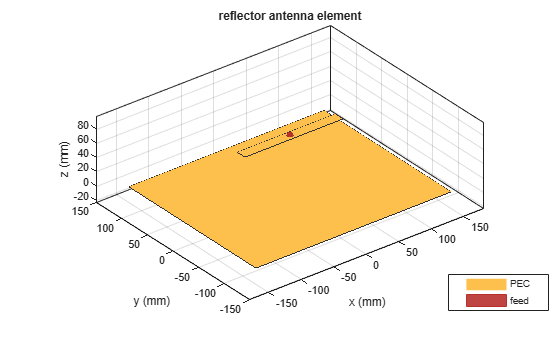Create a reflector backed dipole antenna using a dielectric substrate 'FR4'.

```d = dielectric('FR4'); di = dipole('Length',0.15,'Width',0.015, 'Tilt',90,'TiltAxis','Y'); rf = reflector('GroundPlaneLength',30e-2, 'GroundPlaneWidth',25e-2, ... 'Spacing',7.5e-3,'Substrate',d); rf.Exciter = di; show(rf)```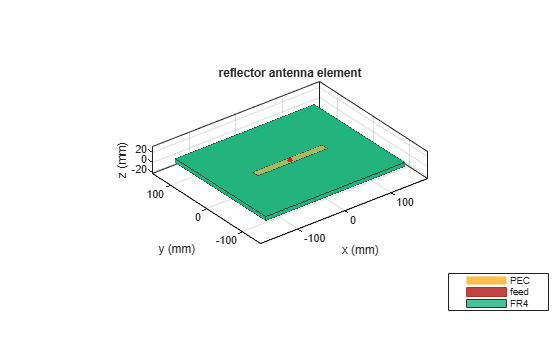Plot the radiation pattern of the antenna at a frequency of 1 GHz.

```figure pattern(rf,1e9)```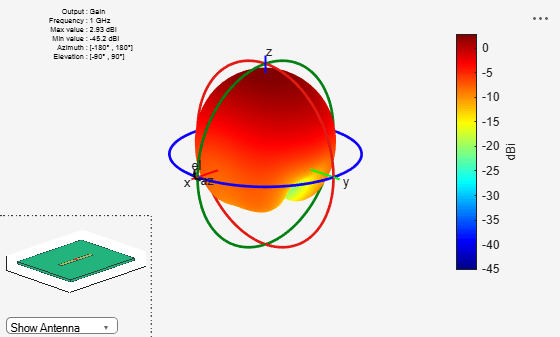Create a reflector backed dipole that has infinite length, 25 cm width and spaced 7.5 cm from the dipole for operation at 1 GHz.

```d = dipole('Length',0.15,'Width',0.015, 'Tilt',90,'TiltAxis',[0 1 0]); rf = reflector('GroundPlaneLength',inf, 'GroundPlaneWidth',25e-2,... 'Spacing',7.5e-2); rf.Exciter = d```
```rf = reflector with properties: Exciter: [1x1 dipole] Substrate: [1x1 dielectric] GroundPlaneLength: Inf GroundPlaneWidth: 0.2500 Spacing: 0.0750 EnableProbeFeed: 0 Conductor: [1x1 metal] Tilt: 0 TiltAxis: [1 0 0] Load: [1x1 lumpedElement] ```
`show(rf)`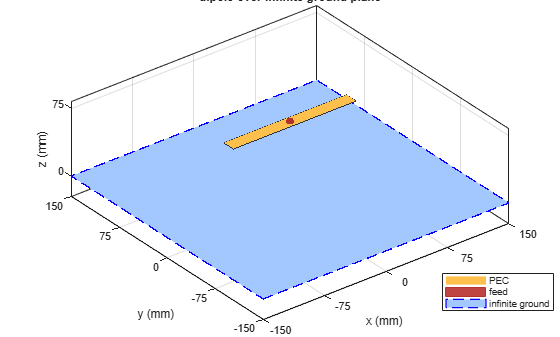Compare the gain values of a dipole antenna in free space and dipole antenna on a substrate.

Design a dipole antenna at 1 GHz.

```d = design(dipole,1e9); l_by_w = d.Length/d.Width; d.Tilt = 90; d.TiltAxis = [0 1 0];```

Plot the radiation pattern of the dipole in free space at 1GHz.

```figure pattern(d,1e9);```Use FR4 as the dielectric substrate.

`t = dielectric('FR4')`
```t = dielectric with properties: Name: 'FR4' EpsilonR: 4.8000 LossTangent: 0.0260 Thickness: 0.0060 For more materials see catalog ```
```eps_r = t.EpsilonR; lambda_0 = physconst('lightspeed')/1e9; lambda_d = lambda_0/sqrt(eps_r);```

Adjust the length of the dipole based on the wavelength.

```d.Length = lambda_d/2; d.Width = d.Length/l_by_w;```

Design a reflector at 1 GHz with the dipole as the excitor and FR4 as the substrate.

```rf = design(reflector,1e9); rf = reflector('Exciter',d,'Spacing',7.5e-3,'Substrate',t); rf.GroundPlaneLength = lambda_d; rf.GroundPlaneWidth = lambda_d/4; figure show(rf)```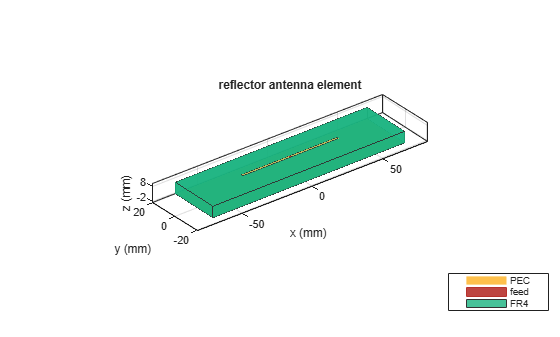Remove the groundplane for plotting the gain of the dipole on the substrate.

```rf.GroundPlaneLength = 0; show(rf)```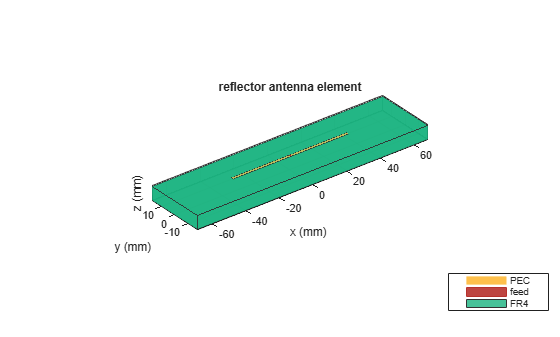Plot the radiation pattern of the dipole on the substrate at 1 GHz.

```figure pattern(rf,1e9);```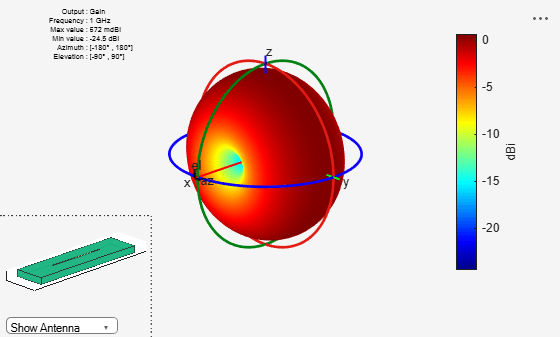Compare the gain values.

• Gain of the dipole in free space = 2.11 dBi

• Gain of the dipole on substrate = 1.93 dBi

Create a rectangular array of the bowtie antennas.

`b = bowtieTriangular('Length',0.05)`
```b = bowtieTriangular with properties: Length: 0.0500 FlareAngle: 90 Conductor: [1x1 metal] Tilt: 0 TiltAxis: [1 0 0] Load: [1x1 lumpedElement] ```
`rectArr = rectangularArray('Element',b,'RowSpacing',0.18,'ColumnSpacing',0.18)`
```rectArr = rectangularArray with properties: Element: [1x1 bowtieTriangular] Size: [2 2] RowSpacing: 0.1800 ColumnSpacing: 0.1800 Lattice: 'Rectangular' AmplitudeTaper: 1 PhaseShift: 0 Tilt: 0 TiltAxis: [1 0 0] ```

Create a rectangular array with reflector backing structure.

`ant = reflector('Exciter',rectArr)`
```ant = reflector with properties: Exciter: [1x1 rectangularArray] Substrate: [1x1 dielectric] GroundPlaneLength: 0.2000 GroundPlaneWidth: 0.2000 Spacing: 0.0750 EnableProbeFeed: 0 Conductor: [1x1 metal] Tilt: 0 TiltAxis: [1 0 0] Load: [1x1 lumpedElement] ```
`show(ant)`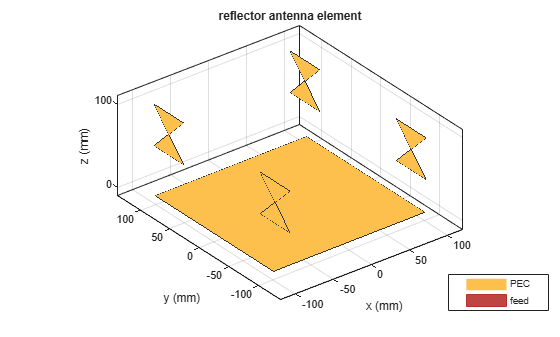Balanis, C.A. Antenna Theory. Analysis and Design, 3rd Ed. New York: Wiley, 2005.